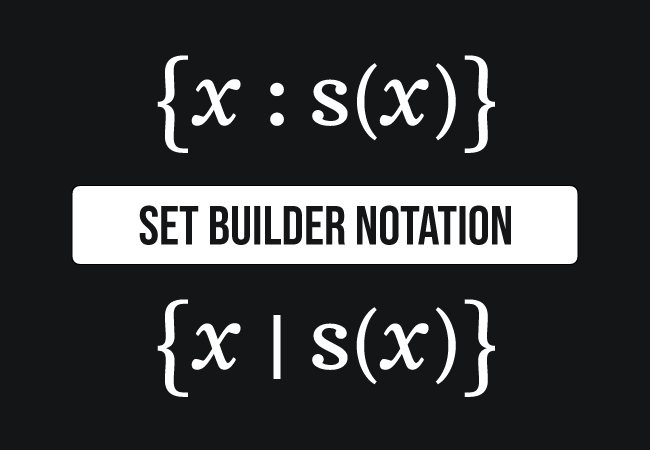# Set-builder notation

A notation that expresses a set constructively by defining the properties of members (or elements) in a mathematical rule is called the set-builder notation.

## Introduction

Mathematics is working on the basis of rules or formulas. So, the idea had come to express a set constructively in a special mathematical notation by defining the properties of elements (or members) in a formula. Hence, the constructive representation of a set in a mathematical rule is called the set-builder notation.The set-builder notation consists of the following three parts.

1. A variable
2. A Separator
3. A formula

Now, let’s learn each part in the set-builder form understandably.

### A variable

A variable is used to represent each member (or element) in a set.

### A separator

A colon $(:)$ or vertical bar $(|)$ is used to separate the formula from the variable in the notation. The separator is read as “such that” or “for which” or “with the property that”.

### A rule

A set is usually defined by considering one or more common properties of the elements. It can be expressed by a logical predicate (or formula).

### Notation

A set-builder notation of any set can be expressed in anyone of the following two forms.

$(1).\,\,\,$ $\Big\{x \,\,:\,\, s(x)\Big\}$

$(2).\,\,\,$ $\Big\{x \,\,\,\,|\,\,\,\, s(x)\Big\}$

In this set, the curly brackets represent a set. Inside the curly braces, a variable, a separator and a logical predicate are written one after one for expressing a set in a mathematical form. In the above form, $x$ is a variable, colon or vertical bar is a separator and $s(x)$ is a formula that the one or more common properties of the objects (or elements).

The roster notation is a simple notation for expressing a set in mathematical form but it has some limitations. Hence, the concept of set-builder notation is introduced in set theory.

#### Examples

Let’s learn the concept of set-builder form from some understandable examples.

The numbers, which are greater than $5$ in set-builder notation.

$(1).\,\,\,$ $A$ $\,=\,$ $\Big\{x \,:\, x \,>\, 5\Big\}$
$(2).\,\,\,$ $A$ $\,=\,$ $\Big\{x \,\,\,|\,\,\, x \,>\, 5\Big\}$

The set $A$ is read as the set of all $x$ such that $x$ is greater than zero.

The numbers, which are greater than or equal to $8$ in set-builder notation. In this example, the type of number is defined. Hence, the set $B$ is written in the following form.

$(1).\,\,\,$ $B$ $\,=\,$ $\Big\{x \,:\, x \,∈ \, N \,\,and\,\, x \,\ge\, 8\Big\}$
$(2).\,\,\,$ $B$ $\,=\,$ $\Big\{x \,\,\,|\,\,\, x \,∈ \, N \,\,and\,\, x \,\ge\, 8\Big\}$

The set $B$ is read as the set of all $x$ such that $x$ is a natural number and the value of $x$ is greater than $8$. The set $B$ is also written in the following form simply.

$(3).\,\,\,$ $B$ $\,=\,$ $\Big\{x \,∈ \, N \,:\, x \,\ge\, 8\Big\}$
$(4).\,\,\,$ $B$ $\,=\,$ $\Big\{x \,∈ \, N \,\,\,|\,\,\, x \,\ge\, 8\Big\}$

The real numbers, which are less than or equal to $1$ and greater than $4$ in set-builder form.

$(1).\,\,\,$ $C$ $\,=\,$ $\Big\{x \,∈ \, R \,:\, x \,\le\, 1 \,\,\,or\,\,\, x \,>\, 4\Big\}$
$(2).\,\,\,$ $C$ $\,=\,$ $\Big\{x \,∈ \, R \,\,\,|\,\,\, x \,\le\, 1 \,\,\,or\,\,\, x \,>\, 4\Big\}$

The set $C$ is read as the set of all $x$, which are real numbers such that the value of $x$ is less than or equal to one or greater than four.

Latest Math Topics
Jun 26, 2023
Jun 23, 2023

Latest Math Problems
Jul 01, 2023
Jun 25, 2023
###### Math Questions

The math problems with solutions to learn how to solve a problem.

Learn solutions

Practice now

###### Math Videos

The math videos tutorials with visual graphics to learn every concept.

Watch now

###### Subscribe us

Get the latest math updates from the Math Doubts by subscribing us.